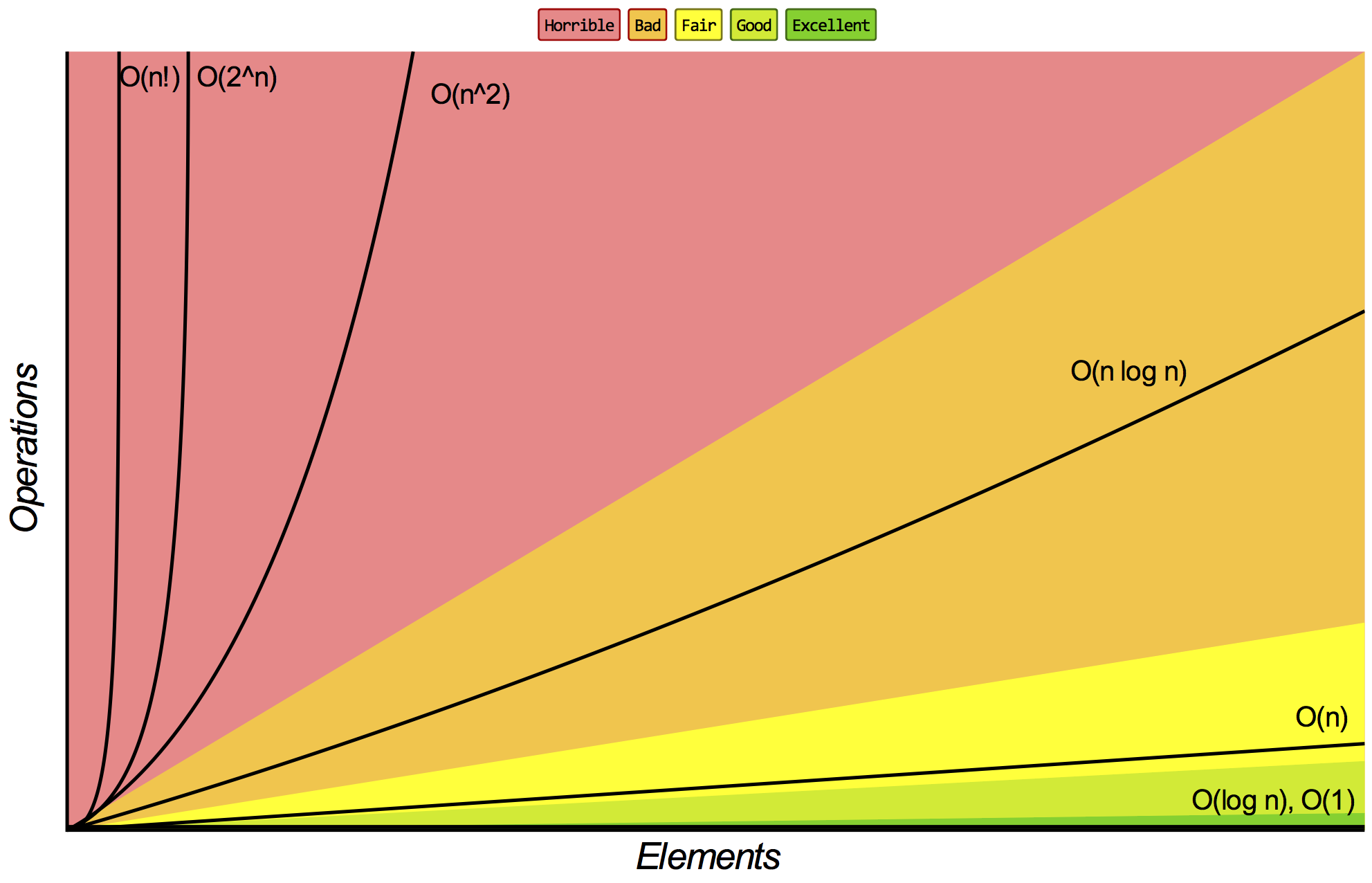Big-O, Big-Omega, and Big-Theta

Daniel Weibel
Created 1 Aug 2017
Last updated 9 Nov 2017
$\newcommand{\O}{\text{O}}$

For general explanations see Summary.

Big-$\O$ vs. Big-$\Omega$ vs. Big-$\Theta$

Big-$\O$

• Upper bound
• Less than or equal: $\leq$

Big-$\Omega$ (Big-Omega)

• Lower bound
• Greater than or equal: $\geq$

Big-$\Theta$ (Big-Theta)

• Big-$\O$ AND Big-$\Omega$
• Tight bound

For an algorithm for which $\O(N)$ is valid, any greater term, e.g. $\O(N^2)$, is also valid (since Big-$\O$ is an upper bound, that is, less than or equal).

For an algorithm for which $\Omega(N)$ is valid, any smaller term, e.g. $\Omega(1)$, is also valid (since Big-$\Omega$ is a lower bound, that is, greater than or equal).

However, in practice, the tightest possible bounds are usually used. That is, the smallest term for Big-$\O$ and the largest term for Big-$\Omega$.

Rules for determining the Big-$\O$/$\Omega$/$\Theta$ term

1. Drop Constants

• $\O(2N)$ becomes $\O(N)$
• Because, no matter how much the constants of e.g. $2\,000\,000 N$ and $2N^2$ differ, with growing input size $N$, the latter term will eventually surpass the former one.
• We are interested in the order of growth only of the running time, and with large $N$, constants and non-dominant terms become relatively insignificant.

2. Drop Non-Dominant Terms

• $\O(N^2 + N)$ becomes $\O(N^2)$
• $\O(5\cdot2^N + 3N^{100})$ becomes $\O(2^N)$
• $\O(A^2 + B)$ stays $\O(A^2 + B)$
• Explanation, see above

3. Different Steps of an Algorithm are Added

• Two successive for loops taking A and B iterations, respectively: $\O(A + B)$

4. Nested Steps in an Algorithm are Multiplied

• Two nested for loops taking A and B iterations, respectively: $\O(A \cdot B)$

5. Use Different Variables for Different Inputs

• For example, if the input consists of two strings of length $n$ and $m$, use two variables $N$ and $M$
• For example, $\O(N + M)$

Base of Logarithm

The base of a logarithm doesn’t matter: log denotes a logarithm of any base.

Base of Exponent

The base of an exponent matters: $\O(2^N)$ and $\O(8^N)$ are different.

Non-Dominant Terms in Exponent

Non-dominant terms in an exponent don’t matter: $2^{N+1}$ becomes $\O(2^N)$.

Constants in Exponent

Constant factors in exponents matter: $\O(8^N)$ and $\O(8^{2N})$ are different.

Common Big-$\O$/$\Omega$/$\Theta$ Terms and Their RelationSource: bigocheatsheet.com

$\O(N^N) > \O(N!) > \O(2^N) > \O(N^2) > \O(N\,log\,N) > \O(N) > \O(log\,N) > \O(1)$

Summary

Big-$\O$/$\Omega$/$\Theta$ notation is used to express complexity bounds of an algorithm.

The term within the Big-$\O$/$\Omega$/$\Theta$ notation denotes a number of steps (time complexity) or used memory units (space complexity).

The variables within the term are are the sizes of the inputs of the algorithm.

What’s the difference between $\O$, $\Omega$, and $\Theta$?

$\O$, $\Omega$, and $\Theta$ defines what the term inside the notation means (see here):

• $\O$: the real value is less than or equal to the notation term (the term is an upper bound)
• $\Omega$ the real value is greather than or equal to the notation term (the term is a lower bound)
• $\Theta$ the real value is equal to the notation term (the term is a tight bound)

How to understand the term within Big-$\O$/$\Omega$/$\Theta$?

However, the Big-$\O$/$\Omega$/$\Theta$ term is not an exact term. Its purpose is to indicate an order rather than an exact value.

That’s why we apply the rules listed here to the Big-$\O$/$\Omega$/$\Theta$ term.

For example, if the tightest upper bound for an algorithms would really be $2N^2$, the Big-$\O$ term is just $\O(N^2)$. Now, if the maximum number of steps of this algorithm is exactly $2N^2$, the algorithm is still in $\O(N^2)$ (even though $2N^2 \leq N^2$ is false).

Conclusion: don’t take the Big-$\O$/$\Omega$/$\Theta$ literally, but just as an indication of order or category.